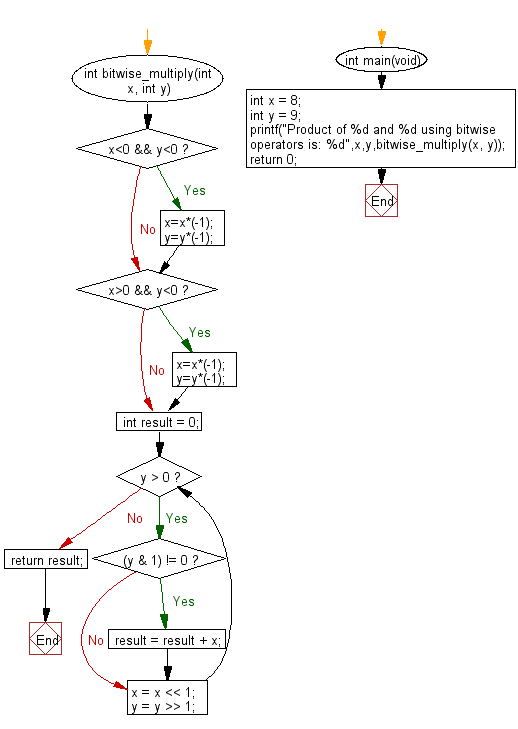﻿ C Program: Multiply two numbers using bitwise operators - w3resource

# C Exercises: Multiply two numbers using bitwise operators

## C Programming Mathematics: Exercise-27 with Solution

Write a C program to multiply two numbers using bitwise operators.

Example:
Input: int x = 8
int y = 9
Output: Product of 8 and 9 using bitwise operators is: 72

Sample Solution:

C Code:

``````#include <stdio.h>
#include <math.h>

int bitwise_multiply(int x, int y)
{
if (x<0 && y<0)
{
x=x*(-1);
y=y*(-1);
}
if (x>0 && y<0)
{
x=x*(-1);
y=y*(-1);
}
int result = 0;
while (y > 0)
{
if ((y & 1) != 0)
result = result + x;
x = x << 1;
y = y >> 1;
}
return result;
}
int main(void)
{
//int x = 12;
//int y = 55	       ;
int x = 8;
int y = 9;      ;
printf("Product of %d and %d using bitwise operators is: %d",x,y,bitwise_multiply(x, y));
return 0;
}
```
```

Sample Output:

```Product of 8 and 9 using bitwise operators is: 72
```

Flowchart:C Programming Code Editor:

Improve this sample solution and post your code through Disqus.

What is the difficulty level of this exercise?

Test your Programming skills with w3resource's quiz.

﻿